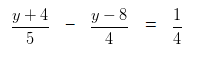# Linear equations in one variable Class 8 WorksheetsQ.1) Ten decreased from a number equals to 20 . Find the number ?
a) 33
b) 29
c) 30

Q.2) The number is subtracted from 23 the result is 10 less than twice the number. Find the number?
a) 10
b) 12
c) 11

Q.3) If the number is multiplied by 7 and 6 is subtracted from the product, the result is 15. What is the number ?
a) 2
b) 3
c) 4

Q.4) The sum of three consecutive number is 99 . What are the numbers ?
a) 31 , 32 , 34
b) 31 , 32 , 33
c) 32 , 33 , 34

Q.5) A man pays 5 paisa tax on Rupee 1 of income earned. If the total tax paid by him is Rupees 1500 , Then how much amount did he earn ?
a) 30002
b) 30000
c) 300000

Q.6) The sum of three consecutive odd natural number is 45 Then find the numbers ?
a) 14 , 17 , 19
b) 13 , 15 , 17
c) 13 , 15 , 18

Q.7) The sum of two consecutive even numbers is 30 . What are the numbers ?
a) 12 and 14
b) 14 and 16
c) 12 and 13

Q.8) The sum of two consecutive odd numbers is 56 . What are the three numbers ?
a) 22 and 34
b) 31 and 25
c) 27 and 29

Q.9) If 3 is added to twice a certain number, the sum is 35. What is the number?
a) 12
b) 18
c) 16

Q.10) Seven times a certain number diminish by 6 , equals 43 . Find the number ?
a) 4
b) 6
c) 7

Q.11) Thrice the number is increased by 12 equals 30 . What is the number ?
a) 8
b) 6
c) 12

Q.12) Half of a number is 10 more than One-seventh of the number . What is the number ?
a) 29
b) 28
c) 27

Q.13) a number is as much as less than 255 as it is greater than 135 . What is the number ?
a) 193
b) 195
c) 197

Q.14) if 4 – 5 ( a – 8 ) = a + 14 ,find the value of a ?
a) 2
b) 5
c) 4

Q.15) What is the value of y if,a) y = 49
b) y = 51
c) y = 57

Q.16) The four positive consecutive integers are such that one-third of the smallest of these numbers exceeds one-ninth of the largest by 9 ? What are the four positive consecutive integers ?
a) 42 , 44 , 46 , 47
b) 42 , 43 , 44 , 45
c) 42 , 43 , 45 , 46

Q.17) The measure of an angle of triangle is equal to the sum of the measure of the remaining two angles. If the ratio of the measures of the remaining two angles is 7 : 8 , then find all the three angles of a triangle ?
a) 42˚, 48˚, 90˚
b) 32˚, 53˚, 95˚
c) 37˚, 43˚, 100˚

Q.18) The sum of two numbers is 77 and their ratio is 4 : 3 . The number are ?
a) 44 , 33
b) 48 , 29
c) 48 , 29

Q.19) Anuj is currently 7 years older than Pramod . If the sum of their ages is 33 years. What is the age of Anuj and Pramod ?
a) Pramod’s age = 13 years , Anuj’s age = 20 years
b) Pramod’s age = 16 years , Anuj’s age = 17 years
c) Pramod’s age = 18 years , Anuj’s age = 15 years

Q.20) Make two parts of 135 such that one part is four-fifth of the Second part . Calculate both the parts ?
a) 60 and 67
b) 65 and 80
c) 60 and 75

Q.1) c. 30
Q.2) c. 11
Q.3) b. 3
Q.4) c. 32 , 33 , 34
Q.5) b. 30000
Q.6) b. 13 , 15 , 17
Q.7) b. 14 and 16
Q.8) c. 27 and 29
Q.9) c. 16
Q.10) c. 7
Q.11) b. 6
Q.12) b. 28
Q.13) b. 195
Q.14) b. 5
Q.15) b. y = 51
Q.16) b. 42 , 43 , 44 , 45
Q.17) a. 42˚, 48˚, 90˚
Q.18) a. 44 , 33
Q.19) a. Pramod’s age = 13 years , Anuj’s age = 20 years
Q.20) c. 60 and 75